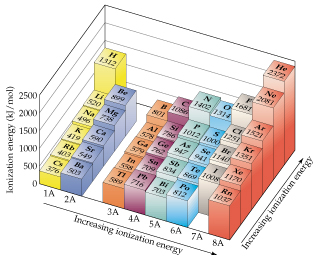# Problem: One way to measure ionization energies is photoelectron spectroscopy (PES), a technique based on the photoelectric effect. In PES, monochromatic light is directed onto a sample, causing electrons to be emitted. The kinetic energy of the emitted electrons is measured. The difference between the energy of the photons and the kinetic energy of the electrons corresponds to the energy needed to remove the electrons (that is, the ionization energy). Suppose that a PES experiment is performed in which mercury vapor is irradiated with ultraviolet light of wavelength 58.4 nm. The kinetic energy of the emitted electrons is measured to be 10.75 eV. What is the first ionization energy of Hg, in kJ/mol?

🤓 Based on our data, we think this question is relevant for Professor Ratliff's class at USF.

###### FREE Expert Solution

We’re asked to determine the first ionization energy (in kJ/mol) of mercury, Hg, given the kinetic energy of the emitted electron and wavelength during PES.

Recall that the ionization energy corresponds to the energy needed to remove an electron, it is the difference between the energy of the photons and the kinetic energy of the electrons.

This means that we can solve for the first ionization energy, using the equation:

$\overline{){\mathbf{I}}{\mathbf{.}}{\mathbf{E}}{\mathbf{.}}{\mathbf{=}}{{\mathbf{E}}}_{{\mathbf{photon}}}{\mathbf{-}}{\mathbf{K}}{\mathbf{.}}{\mathbf{E}}{{\mathbf{.}}}_{{\mathbf{electron}}}}$

Also recall that the energy, E of a photon, is given by the equation:

$\overline{){\mathbf{E}}{\mathbf{=}}{\mathbf{h\nu }}}$

Etotal energy = J
h = Planck’s constant = 6.626x10-34 J
s
ν = frequency = Hz or s-1###### Problem Details

One way to measure ionization energies is photoelectron spectroscopy (PES), a technique based on the photoelectric effect. In PES, monochromatic light is directed onto a sample, causing electrons to be emitted. The kinetic energy of the emitted electrons is measured. The difference between the energy of the photons and the kinetic energy of the electrons corresponds to the energy needed to remove the electrons (that is, the ionization energy). Suppose that a PES experiment is performed in which mercury vapor is irradiated with ultraviolet light of wavelength 58.4 nm.The kinetic energy of the emitted electrons is measured to be 10.75 eV. What is the first ionization energy of Hg, in kJ/mol?